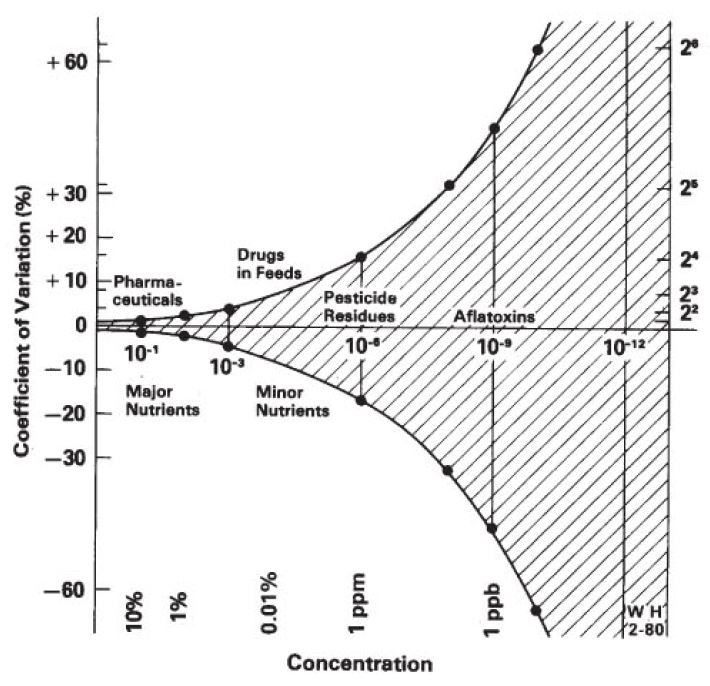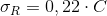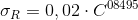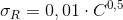• +55 (11) 3767-4109
• This email address is being protected from spambots. You need JavaScript enabled to view it.
• Mon- Fri 8:00 - 17:00

Proficiency programs IPT/LRM offer many evaluations of chemical analyses in different types of materials.  It's relatively common that during the rounds of the programmes, some participants stand in doubt about an important issue.  Why are grouped results generated by different methods, carried out by means of different analytical techniques.  How is it possible to perform the statistical evaluation of these results together, since the methodologies are not identical?

The answer is that the LRM/IPT adopted a general model that allows this grouped approach.  The model used is based on the Horwitz equation, modified by Thompson.  This model is Dr. William Horwitz, who first noticed that there was a relationship between the variance of the results between laboratories and the concentration of the analyte, and that this relationship not depended on the chosen methods of analysis or the analytes.This template is very useful as it provides an estimate of variability for the chemical properties, which depends exclusively on the concentrations of the analytes, regardless of the method of analysis in particular.  This approach estimates a maximum variability, and is considered suitable by the ISO 13528:2015, that guides the statistical calculations for the standard NBR ISO IEC 17043, we adopted.

The model is composed of 3 empirical equations, which are employed according to the concentration of analyte present in the samples of the program.  Important to remember that Horwitz relations are presented as a function of the fraction by weight C.

When C <1, 2 x 10-7 , i.e. when the concentration of the analyte is less than 0.12 mg/kg, or 0.12 ppm, we use the following equation to represent the interlaboratory standard deviation:When 1, 2 x 10-7 C <<0.138 (merger between 0.12 mg/kg and 13.8%), use the following equation to represent the standard deviation:When C is greater than 0.138 (concentration>13.8%), use the following equation to represent the standard deviation:So, the model provides the variability, represented by standard deviations obtained in the application of one of the equations presented, and these standard deviations are used in the composition of the target standard deviation Round proficiency program, saying so simplified and summarized.

Particularly, do not consider this an ideal solution. In theory, the best approach would be to assess the results of each specific method, as each method has a reproducibility of its own, but in practice, it is necessary that there is also a sufficient number of participants per method, so this approach is statistically reliable.

Most of the time, the numbers of results for each individual method are not large, and for this reason we have adopted this methodology of grouping of results, where appropriate, as it is relatively safe and reliable in most situations, Apart from being provided for in international standardization and practiced by most traditional providers around the world.